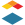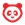cancel
Showing results for
Show  only  | Search instead for
Did you mean:

# Scientific Notation in Formula Questions Redux

I would like to add my thanks to Canvas for incorporating scientific notation in numerical answer questions, but following on this I would also like to resuscitate an earlier idea that is currently in cold storage to incorporate scientific notation in formula questions as well. For all the same arguments that were presented to include it in numerical answer questions, it is essential to have it in formula questions too, and I'm hoping that having done it in the former, it won't be too difficult for Canvas to do it in the latter.

I won't reiterate those arguments here (I'm happy to do so if needed), but rather make a personal plea. I am in the process of migrating hundreds of calculated formula questions from Blackboard to Canvas, and the vast majority of those require scientific notation and precision (number of significant figures). While I am able to tweak a few of them to work with standard notation (asking for the answer in GHz rather than Hz, changing it from 1.23e+11 Hz to 123 GHz, for example), there are many of these questions which simply will not work in Canvas without scientific notation.Instructure

I was able to find two relevant ideas with helpful discussion in the comments (both in Cold Storage so you will need to join that space to view them):

@danderso , if this is an urgent need you may want to also work through your local support and Canvas admin who has access to your institution's Customer Success Team to see if there are any other options available beyond the feature idea process.New Member

I put a similar idea up and was directed to move my comment over to this idea thread.

Answers should be have the option to be in scientific notation format with a user-defined number of significant digits, not just 4 digit decimal. This is a huge problem in running chemistry or physics courses that can have very large or very small values. eg currently in a chemistry equation generating solutions gives 80% with the final answer rounded to 0.0003, when in reality there is a world of difference between 0.000266 and 0.000341. It would be preferable to have the students submit the answer "2.66E-4" or "2.66*10^-4"New Member

I really appreciate the inclusion of formula questions (took canvas from D+/C- to B-/B) but being able to define the number of digits, i.e. scientific notation, for answers smaller than 4 decimal places and larger than 1,000 for both the answers and the variable definitions would be extremely useful. I've used the work-arounds noted above.New Member

As a chemistry teacher who teaches scientific notation, I feel that it is imperative that numerical information can be entered in scientific notation format.  It will make students less frustrated and make grading easier.New Member

I know everyone who uses canvas doesn't need scientific notation, but for those of us that do, it's critical.New Member

Please do this ASAP - our whole university is moving to Canvas (20000 students; over 4000 science students who will need to use very small numbers) from a combination of moodle and blackboard and whilst having quizzes built in is great the lack of ability to use scientific notation in formula questions is already becoming an issue as an early adopter !

Also what about units ? In moodle u could have both a calc figure and a units drop down - i don't see that here 😞

Don't get me wrong I think Canvas is a great step up for us but wanted to iron out issues !New Member

Scientific notation would be a great improvement but it would also need an area to choose a prefix as a drop-down along with a defined unit. I also feel that it would be helpful to define a formula equation that can have two answers submitted for complex systems. Alternatively a formula with variables that can be used over multiple questions.Community Team

Please take a look at the Canvas Release Notes (2019-06-01)‌: Numeric Question Scientific Notation Responses.New Member

I've looked at the Canvas Release Notes (2019-06-01)‌.  I would like to see the formula question type include the ability to indicate how many significant digits are required, as the numerical question now does in quizzes.next.  As previous posts have noted, there is a need for incorporating standard prefixes and units as part of the answer.New Member

Related post: Significant Figures, too!New Member

For pure sciences and engineering sciences, the ability to use scientific notation in answers and in formulae is critical.  Furthermore, it is essential to bracket quiz answers with an upper and lower limit because roundoff errors can cause students who correctly solve a problem to enter different but acceptably correct answers.Community Participant

I am curious what the status of sci notation is. Right now I am under the impression that in the old quiz there is no scientific notation. In the New quiz there is in a numerical answer but not the formula question. The formula question I thought did work a few months ago but is not now.

Is this correct? As I madly try to make quizes i won't have to rewrite.New Member

I would like to add that having scientific notation as a valid way to represent numbers in the formula question type is pretty critical for my courses (both in the question, and in the answers).  What I'm finding currently is that numbers can be inputted using scientific notation as answers, but the range is quite limited.  It would be ideal if there was no truncation between 1E-150 to 1E150.  Additionally, being able to put an error tolerance on solutions represented by numbers in scientific notation is also necessary.Community Member

It is very important to support exponential notation in formulas themselves as well as in answers. This is particularly important in physics where we may have large numbers in conversions, and I'm sure it is important in chemistry (Avogadro's number, anyone?) and engineering too.  Currently the Canvas formula will reject "1.0e9" as an invalid variable.  It is inconvenient and error prone to have to write 1000000000.Community Participant

I originally posted this problem in the user guide comments and was told to post it here, so I am copying it over mostly verbatim.

I noticed that display as scientific notation removes the ability to apply a margin of error to the questions, which is a huge problem because in testing I found that an answer of 1.2 would be marked incorrect against a correct answer of 1.20 and vice versa.  The margin of error needs to be added to scientific notation otherwise it is essentially useless because.

Alternatively, or additionally, the ability to separately grade sig figs would be very useful. Maybe a button that provides the option to apply a point or percent deduction.

The mechanism for entering scientific notation is also problematic.  Most of us are accustomed to using the “E” format in calculations from Excel and calculators, such as 1.20E-2, but the formula question uses 1.20*10^-2, which is really convoluted.  The formula questions need to accept both or the easier “E” format, or provide a clear indication of expectations.Community Member

I too would like Canvas to support scientific notation / "E" format. Designing formula questions is currently a rather annoying experience. You have to tweak the question and/or the unit of the answer such that it is larger than 0.001 or so. A work around I have used is to ask for calculated diffusivity in air in the unit cm2/s rather than the SI-unit m2/s. That workaround does not work for e.g. a  membrane resistance of 2E12 m^(-1)

Interpreting scientific notation is pretty basic stuff so I can't really see what seems to be the problem in implementing it in Canvas. In many engineering disciplines it is really tricky to design reasonable exercises and exam tasks without using scientific notation in the question and in the answer.Community Member

I agree that writing 6.02E23 is less convoluted than 6.02*10^23; however, this is a minor point compared to the following:

In chemistry really big (Avogadro's Number, 6.02E23) and really small numbers (solubility product constants - Ksp for bismuth sulfide is 1.6E-72) are routinely used.   Thus, it is essential that for formula questions that a wide range of power of tens be allowed and that a +/- margin of error be included for answers reported in scientific notation.

Significant figures are important in chemistry as well and very few LMS programers/decision makers seem to care about the importance of trailing zeros.

Please don't exclude the needs of science/math/engineering faculty that need to impart important concepts to our students.New Member

The chemistry teachers at my school continue to use moodle because it has significant figures and units for numerical/formulaic problems.  It doesn't possible in new quizzes.  And I would get more buy in from my science and math teachers with this ability.Community Member

So, scientific notation is acceptable in Canvas Quizzes now, but this seems to be now required.  If the answer is 1.24*10^-2 then 0.0124 is marker as WRONG.   This is absolutely absurd.   If Canvas can do math, then it should be able accept alternative ways of expressing the same thing.Community Member

I'd wish that there were 3 options provided in a numeric input.

Choice 1:  Only accept the answer in scientific notation.  The reason for this would be if the instructor wished to ascertain the ability of a student to write a number in scientific notation (e.g.,  Write 0.00123 in scientific notation).

Choice 2:  Only accept the answer in "decimal/standard notation".  The reason for this would be if the instructor wished to ascertain the ability of a student to convert a number written in scientific notation into standard notation (e.g.,  Write 1.23x10⁻⁴ in standard notation).

Choice 3:  Accept the answer in either scientific notation or standard notation when you are not trying to evaluate whether or not a student could write their answer in either type of notation. Choice 3 would address  comment.

Since my Choice 3 is not an option, to circumvent issues with grading, I clearly write in the instructions:  Report your answer in standard notation unless otherwise informed.